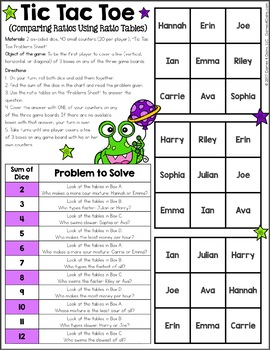# 6th Grade Math Games: Ratios, Proportions, & Unit Rates {6.RP.1, 6.RP.2, 6.RP.3}Subject
Resource Type
Common Core State Standards
Product Rating
File Type

PDF (Acrobat) Document File

Be sure that you have an application to open this file type before downloading and/or purchasing.

19 MB|72 pages
Share
Product Description

These games make 6th grade ratios and proportional relationships standards so much fun to practice! This 6th Grade Ratios, Rates, and Proportions Games Pack contains 30 fun and engaging printable board games to help students to practice using ratios, finding equivalent ratios, calculating rates, calculating percents and percents of a quantity, converting measurements, and much more!

This pack includes 30 ENGAGING games that cover the following 6th grade math skills:

Understanding Ratios and Equivalent Ratios:

• Match pictures to a given ratio (written as 2:3 or 2 to 3)

• Match pictures to a given ratio (written as a fraction)

• Write ratios in three forms for given information

• Find equivalent ratios

• Fill in the missing values in equivalent ratio tables

• Use equivalent ratios to solve basic problems

• Use equivalent ratios to solve advanced problems

• Compare ratios using ratio tables

• Plot pairs of equivalent ratios on the coordinate plane

Solving Problems with Unit Rate:

• Find the unit rate from a given scenario

• Find the unit rate from a given ratio

• Find the unit price

• Compare two scenarios using unit rates

• Use unit rates to solve problems

• Solve problems with distance, time, and rate

Calculating Percents:

• Match models to percents, fractions, decimals, and ratios

• Write percents as fractions and decimals

• Write fractions and decimals as percents

• Calculate the percent when given the part and the whole

• Calculate the part when given the percent and the whole

• Calculate the whole when given the percent and a part

• Calculate percent discount

Converting Measurement Units:

• Convert units of time

• Convert units of length (customary)

• Convert units of length (metric)

• Convert units of mass (customary)

• Convert units of mass (metric)

• Convert units of capacity (customary)

• Convert units of capacity (metric)

These games support the following Common Core standards: 6.RP.A.1, 6.RP.A.2, 6.RP.A.3, 6.RP.A.3.A, 6.RP.A.3.B, 6.RP.A.3.C, and 6.RP.3.D.

These games are so simple to use and require very minimal prep - just print, grab some basic materials, and play! They are perfect to use in math centers or as extension activities when students complete their work!

Included with this 6th Grade Ratios, Rates, and Proportions Games Pack:

• Instructions for play

• 30 printable games

• Answer keys for all of the games

Want to learn more about the games included? Check out the preview file above.

~~~~~~~~~

You might also like these other 6th grade math resources by Games 4 Gains:

6th Grade Operations Games Pack

Ratio Word Problems Scavenger Hunt Cards

Unit Rates Word Problems Logic Puzzles

Converting Fractions, Decimals, Percents "Squares" Games

Percents Word Problems Coloring Cards

Percent Discount Shopping Bingo

6th Grade Jeopardy Math Review Game

~~~~~~~~~

Customer Tips:

We love to hear what you think! Please leave your feedback on this resource to earn credit points to save money on future purchases!

Click on the green ★ above to follow my store to get notifications of new resources, sales, and freebies!

Connect with me on social!

~~~~~~~~~

Created by Brittney Field, © Games 4 Gains, LLC.

This purchase is for single classroom use only. Sharing this resource with multiple teachers, an entire school, or an entire school system is strictly forbidden. Multiple licenses are available at a discount.

Total Pages
72 pages
Included
Teaching Duration
N/A
Report this Resource
Reported resources will be reviewed by our team. Report this resource to let us know if this resource violates TpT’s content guidelines.